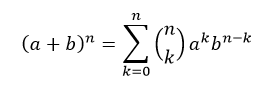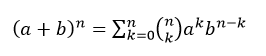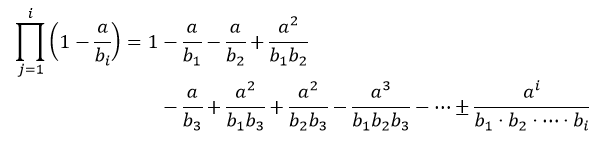# More on Equation Numbering

The book Creating Research and Scientific Documents using Microsoft Word gives a method for numbering equations that works with all versions of Word from Word 2007 on and has automatic renumbering of the equations and the references to the equations. Those are very attractive features. The approach inserts a center tab before the equation and a right tab before the equation number. This works perfectly for simple equations, but there are some problems for more complicated equations. The problems could in principle could be solved with modifications to Word, but not in a backward compatible way. This post explains the problems and possible solutions.

The problems occur because the presence of tabs on the line outside the math zone makes Word think the math zone is an inline math zone and therefore uses inline math typography instead of display math typography. For inline typography, summation limits appear as subscripts and superscripts instead of being displayed above and below. Also fraction numerators and denominators are squeezed down to script level 1. A fix in Word would be to treat a math zone ensconced between such tabs as a display math zone. Such a fix wouldn’t require file format changes. For example, with inline math typography the display-math equationlooks likeThe relatively compressed format is desirable inline so that a paragraph isn’t spaced too far apart. But it doesn’t look as nice as the display math version.

A second problem is that if an equation is too long to fit in the allotted space, it wraps using inline protocol and typically leaves the equation number centered instead of flushed right. Nice wrapping can be obtained using an equation array as discussed below.  A minor inconvenience with the center and flush-right tabs is that if you change the margins, you need to change the center and right tabs as well in order to keep the equation centered and the equation number flushed to the right. This problem would be fixed if Word added center-width and right-margin tabs that derive their positions from the current display width instead of from fixed values on the ruler. Adding two new kinds of tabs would change the tab-type enumeration in files.

If you use the three-cell table approach of Dong Yu, these problems don’t occur and the approach works with Word 2007 on. You can have numbered equations with nice wrapping like(1.1)

The first and third cells have the identical, small widths. The second cell is wide and contains the equation. The third cell contains the equation number. Or you could put the equation numbers in the first cell if your publisher wants equation numbers to be on the left (pretty rare). The three-cell table approach can be used instead of the center and right tabs, while using the approach of the book for formatting and referencing the equation numbers. Chapter 5 of the book discusses using tables to align figures in nice ways and tables are used extensively in HTML web pages to achieve special alignments. In fact, a three-cell HTML table is used here in this post to flush the equation number to the right.

In principle, the Office math handlers offer better approaches to equation numbering than using center and right tabs. An earlier post describes such approaches. The main glitch with the more elegant equation numbering approach using the Page/Table Services math handler is that Word needs some changes to utilize the facility.

Meanwhile an intermediate approach was quietly incorporated into OneNote 2013 and hopefully will show up in other apps pretty soon. This approach uses a special feature of the equation array, which flushes an equation number to the right margin. You enter a numbered equation by typing the equation followed by ‘#’ followed by the equation number and then an Enter key as described in Section 3.21 of the math linear format paper. The # is a code that triggers insertion of an equation array with a # argument separator. The text that follows the separator is flushed to the right of the page. This allows the equation to remain in display mode and to track margin changes automatically. Furthermore, by using a nested equation array with line breaks and appropriate aligning and spacing &’s, you can get arbitrary inter-equation alignments as explained in the equation-array post. The numbering and cross referencing can be accomplished using the techniques explained in Chapter 6 of the book. Once you have one equation number in place, all you need to do is to copy that number to generate appropriately numbered equations elsewhere in the document and bookmark them for cross referencing purposes. Word’s automatic sequence numbering renumbers the equations as need be (you can fire the update by pressing the F9 key). The equation-number option of the equation array doesn’t require any file format changes, but on unaware apps, it displays the equation number adjacent to the equation instead of flushed right.

The good thing is that there are ways to number equations in Word pretty easily, although they aren’t as discoverable as other kinds of numbering in Word. For the moment at least, I like using the three-cell table approach with the formatting and referencing methods described in the book. That combination gives you easy equation number updates along with display-math formatting and nice equation line breaks.

1.davidacoder says:

The problem with all of these suggestions is that I can use them when I work on a paper by myself. I don't mind that kind of tweeking (well, actually, I do, but I can do it). But this simply doesn't work with co-authors that are not inclined like that. The whole situation is really sad. Since 2007 there is a great equation editor in Word, but I have yet to use it successfully with any of my co-authors, simply because of this numbering issue. We use MathType, ONLY because of this. I would love to dump it, but I can't, I cannot seriously ask my co-authors to take the kind of steps you describe here. I am still absolutely baffled, how it can happen that such a wonderful feature is shipped for such a long time with such a fundamental flaw that renders it more or less useless for the vast majority of scientific publishing…

I also have given up any hope that this might ever come. You are probably now busy implementing iPad and Metro versions of everything, that will probably keep the office organization busy for the next 2-3 cycles, and we will never see this issue addressed. Sad, sad, sad.

2.Antonio says:

I totally agree with Davidacoder! It is really sad.

3.Peter Jamieson says:

I suspect "OneNote 2010" is a typo and should be "OneNote 2013". If so, worth fixing the text, otherwise people will wonder just how long it might take to get the featueres into the other Office applications 🙂

4.Sebastian Grunert says:

I would like to see a manual switch to change the layout of formulas between inline and display mode. When I create worksheets for school I need this capability. For example:

a.) formula 1                 b.) formula 2.          c.) formula 3

d.) formula 4.                e.) formula 5.          f.)  formula 6

Sure, I could make a table for it. But with tabstops and the ability to change between inline and display mode manually would be easier.

5.MurrayS3 says:

Re the support for <equation>#<equation number> support in OneNote, it was enabled in OneNote 2013, not 2010. It might have worked in OneNote 2007; I don't have a copy to check. It worked in RichEdit back then. I'll update the post. Thanks

Re display mode with characters on the line not in the math zone, it's a reasonable request as you illustrate. The table does make your case work. I'll put the idea on the wish list. Thanks

6.Mikael Samsøe Sørensen says:

It basically uses the 3-cell table approach as described here. In combination with SEQ-fields to achieve automatic numbering.

Then you can:

press alt+m to insert equation.

press alt+m twice to insert numbered equation (alt+m toggles numbered/unnumbered)

In settings you can choose to have the equation in the left or right side. and choose between two numbering styles (1) or (2.1)

The add-on adds a lot of other functions which you might not need though.

– Latex conversion

– All kinds of Calculations on math expression

7.Erik Esveld says:

@michael  Now that sounds very useful. Im going to check it out right away and will post my first experience with the add in.

8.Michael Osthege says:

Apparently Mikael has set up a Codeplex site in the meantime: http://wordmat.codeplex.com/

9.heinadriaan says:

In Microsoft Word it would be nice to have right aligned automatically generated equation numbers. When referencing to an equation I like to get a list of all equations (in 'professional lay-out'), and then you just have to click on the equation you want to reference.

10.Calchas says:

The problem with the table approach is that it forces a new paragraph. It is very rare to start a paragraph with a mathematical equation; usually the equation is part of a continuous sentence. [The ability to insert commas or other punctuation marks immediately after a display equation would also be handy for this reason.]

This means that special care must be taken with the paragraph spacing so that the "new" paragraph doesn't noticeable to the reader. However I want a 6 pt gap between real paragraphs. So, unless I manually format every paragraph (urgh) I am rather stuck.

As much as I love the equation editor it really doesn't feel like it was intended to be used in serious pieces of work.

11.Princeton University says:

It’s surprising to find on microsoft.com a resource so precious about
equations.
We will note your page as a benchmark for More on Equation Numbering.

We also invite you to link and other web resources for equations
like http://equation-solver.org/ or https://en.wikipedia.org/wiki/Equation.
Thank you ang good luck!